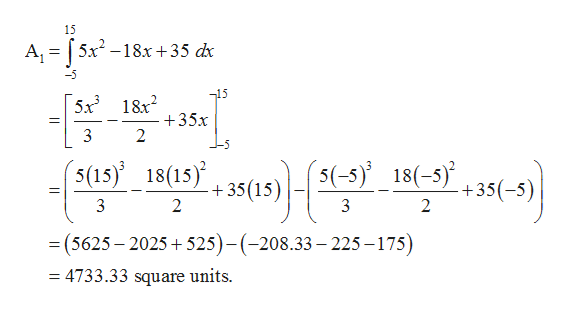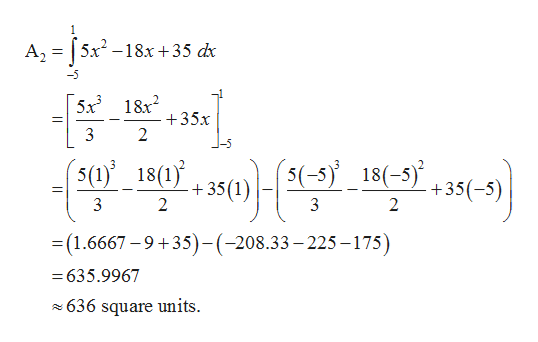# Consider the function g(x)=5x2−18x+35. Find the area under the curve g(x) from x = -5 to x = 15 and then subtract from it the area under the same curve g(x) from x = -5 to x=1. What is the difference?

Question
5 views

Consider the function g(x)=5x2−18x+35. Find the area under the curve g(x) from x = -5 to x = 15 and then subtract from it the area under the same curve g(x) from x = -5 to x=1. What is the difference?

check_circle

Step 1

Area under the curve  g(x) from x = –5 to x = 15help_outlineImage TranscriptioncloseA-jp-2 15 A, =5x-18x +35 dx 15 5x3 18x2 +35x 2 5 (5(-5 18(-3) , 35(-5)| (s(15 18(15) 3 +35 (15) 2 3 2 =(5625-2025 525)-(-208.33 225-175) = 4733.33 square units. fullscreen
Step 2

Area under the curve g(x) from x = –5 ...help_outlineImage Transcriptionclose1 A,=5x-18x +35 dx -5 5x3 1&x2 +35x 2 |-5 5(-518(-535-5) (5(1)18(1j +35 (1 2 3 2 (1.6667-9+35)-(-208.33-225-175) = 635.9967 636 square units fullscreen

### Want to see the full answer?

See Solution

#### Want to see this answer and more?

Solutions are written by subject experts who are available 24/7. Questions are typically answered within 1 hour.*

See Solution
*Response times may vary by subject and question.
Tagged in

### Integration